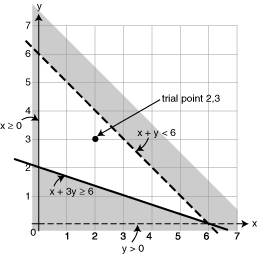Definition: inequality from Philip's Encyclopedia

Mathematical statement that one expression is less, or greater, than another. The symbol > stands for 'is greater than', and < stands for 'is less than'. The symbols ≤ and ≥ stand for 'greater than or equal to' and 'less than or equal to' respectively.

Summary Article: inequality
From The Hutchinson Unabridged Encyclopedia with Atlas and Weather Guide

In mathematics, a statement that one quantity is larger or smaller than another, employing the symbols < and >. Inequalities may be solved by finding sets of numbers that satisfy them. For example, to find the solution set to the inequality 2x + 3 < 19, the inequality can be treated like an equation:

2x + 3 < 19, so 2x < 19 − 3, 2x < 16, and so x < 8.

Therefore, the solution set consists of all values of x less than 8 on the number line. Inequality relationships involving variables are sometimes called inequations.

Graphs of linear inequalitiesGraphs can be used to solve inequalities. For example, this graph shows the sets of inequalities x ≥ 0, y > 0, x + y < 6, and x + 3y ≤ 6:First all 4 lines on the graph are drawn. After drawing the lines x = 0 and y = 0, two sets of coordinates are found that lie on the straight line x + y = 6. The simplest points to find are when x and y are zero: when x = 0 y = 6, and when y = 0 x = 6. These two points are plotted and the line drawn.

Two points are now found that lie on the line x + 3y = 6: when x = 0 y = 2, and when y = 0 x = 6. These two points can be plotted and the line drawn.

Points on the lines y = 0 and x + y = 6 are not included in the region and so the lines are drawn broken.

The lines x = 0 and x + 3y = 6 are both shown as unbroken lines to indicate that the points on the lines are included in the region.

Now the unwanted region is shaded. The unwanted area to the left of the line x = 0 is shaded, and the unwanted area below the line y = 0 is shaded.

To decide what side to shade a sloping line a trial point is required, which must be any point that is not actually on the line. In this example the trial point is (2,3). The trial point (2,3) satisfies the condition x + y < 6, because 2 + 3 = 5, which is less than 6. The unwanted area below the line can, therefore, be shaded.

The trial point (2,3) is also in the region x + 3y ≥ 6, because 2 + (3 × 3) = 11, which is greater than 6. So the unwanted area above the line is shaded.

The required region is left unshaded.

essays

Integers and number line

Maths for Morons Like Us© RM, 2018. All rights reserved.

### Related Articles

##### Full text Article inequality
The Penguin Dictionary of Mathematics

1. A mathematical statement that two expressions are not equal to one another in value. The symbol ≠ is used; for example, x ≠ y means ...

See more from Credo